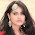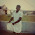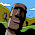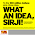## Saturday, 28 December 2013

### CBSE Class 7 - Science - CH 14 - Electric Current and Its Effects (MCQs)

Electric Current and its Effects
(MCQs)Why CFL is better than electric bulb?

Q1: The unit to measure electric resistance is:

(a) Joule
(b) Ohm
(c) Ampere
(d) Volt

Q2: The device used to make or break an electric circuit is:

(a) Resistor
(b) Battery
(c) Switch
(d) Ammeter

Q3: When an electric current passes through a solenoid, it acts as a/an

(a) insulator
(b) electric bell
(c) resistor
(d) bar magnet

Q4:  Which of the following is an essential element in an electric circuit?

(a) switch
(b) connecting wires
(c) voltmeter
(d) ammeter

Q5: Which of the following acts as safety device in electric appliances?

(a) fuse
(b) connecting wires
(c) resistors
(d) battery

Q6: Which of the following does not use magnetic effect of electric current?

(a) electric fan
(b) electric bulb
(c) electric motor
(d) refrigerator

Q7: The magnetic effect of the current was discovered by ________?

(a) Newton
(b) Joule
(c) Oersted
(d) Ohm

Q8: The strength of the magnetic field due to a solenoid can be increased by

(a) decreasing the current strength in the solenoid
(b) increasing the number of turns in the solenoid
(c) by winding solenoid around a plastic rod
(d) all of the above

Q9: Which of the following is the best option while considering the safety of household electric appliances?

(a) We should use wire strip as fuses.
(b) We should use non-ISI marked MCBs
(c) We should use ISI marked MCBs
(d) We should use conductors with high melting point in fuses.

Q10: Compact fluorescent lamps (CFLs) are considered better than ordinary electric bulbs because?

(a) CFLs reduce wastage
(b) CFLs can be fixed in the ordinary bulb holders.
(c) both (a) and (b)
(d) none of the above

1: (b) Ohm
2: (c) Switch
3: (d) bar magnet
4: (b) connecting wires
5: (a) fuse
6: (b) electric bulb
7: (c) Oersted
8: (b) increasing the number of turns in the solenoid
9: (c) We should use ISI marked MCBs
10: (c) both (a) and (b)1.i am an closed circuit witha bright bulb

2.nice question

3.good and nice !!!!!!

4.5.6.This comment has been removed by the author.

7.NICE QUESTIONS TO RECALL THE WHOLE CHAPTER QUICKLY.

8.Good but add more questions

9.I have learnt lots of things about Electronics by these questions thanks

10.11.very nice and useful

12.Thank you good questions, I like it

13.Thanks bro can u plz make more question from sst book class 6 from geography any chapter